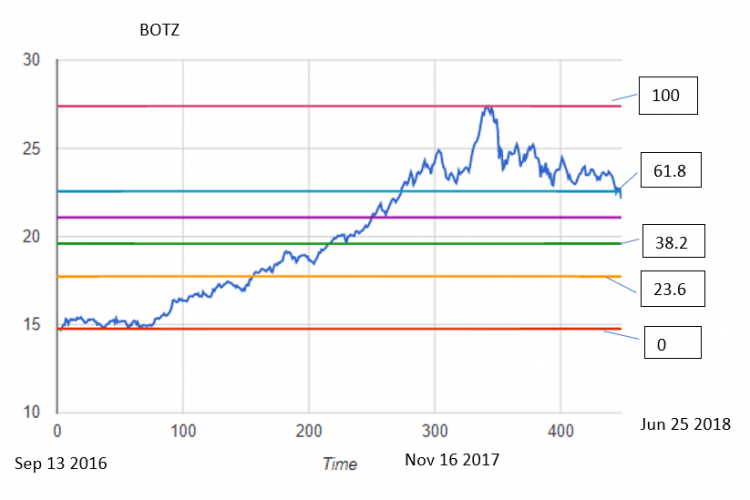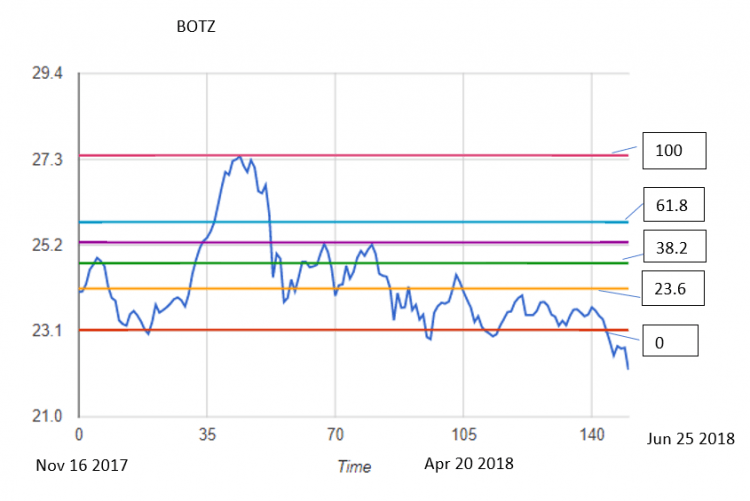# Fibonacci Stock Trading – Using Fibonacci Retracement for Stock Market Prediction

As stated on allstarcharts.com by expert with more than 10 years, Fibonacci Analysis is one of the most valuable and easy to use tools for stock market technical analysis. And Fibonacci tools can be applied to longer-term as well as to short-term. 

In this post we will take a look how Fibonacci numbers can help to stock market analysis. For this we will use different daily stock prices data charts with added Fibonacci lines.Just for the references – The Fibonacci numbers (or Fibonacci sequence), are numbers that after the first two are the sum of the two preceding ones : 0, 1, 1, 2, 3, 5, 8, 13, 21, 34, 55 …

According to “Magic of Fibonacci Sequence in Prediction of Stock Behavior”  Fibonacci series are widely used in financial market to predict the resistance and support levels through Fibonacci retracement. In this method major peak and trough are identified, then it is followed by dividing the vertical distance into 23.6%, 38.2%, 50%, 61.8% and 100%. These percentage numbers (except 50%) are obtained by dividing element in Fibonacci sequence by its successors for example 13/55=0.236. 
Ratio of two successive numbers of Fibonacci sequence is approximately 1.618034, so if we multiply 23.6 by 1.618034 we will get next level number 38.2.

## 5 Fibonacci Chart Examples

Now let’s look at charts with added Fibonacci lines (23.6%, 38.2%, 50%, 61.8% and 100%).

Below are 5 daily the stock market price charts for different stock tickers. Fibonacci line numbers are shown on the right side, and stock ticker is on the top of chart.Botz stock data chart with Fibonacci lines
Here we can see Fibonacci line at 61.8 is a support lineBotz stock data chart with Fibonacci lines

This chart is for the same symbol but for smaller time frame. 23.6 line is support and then resistance lineGE stock data chart with Fibonacci lines
Here are Fibonacci Retracement lines at 23.6 and 61.8 line up well with support and resistance areasGE stock data chart with Fibonacci lines
Fibonacci Retracement 61.8 is support lineT stock data chart with Fibonacci lines
Fibonacci Retracement lines such as 23.6, 38.2, 61.8 line up well with support and resistance areas

## Conclusion

After reviewing above charts we can say that Fibonacci Retracement can indicate potential support and resistance levels. The trend often is changing in such areas. So Fibonacci retracement can be used for stock market prediction.

Do you use Fibonacci tools? Feel free to put in the comments how do you apply Fibonacci retracements in stock trading. Do you find Fibonacci tools helpful or not? As always any feedback is welcome.